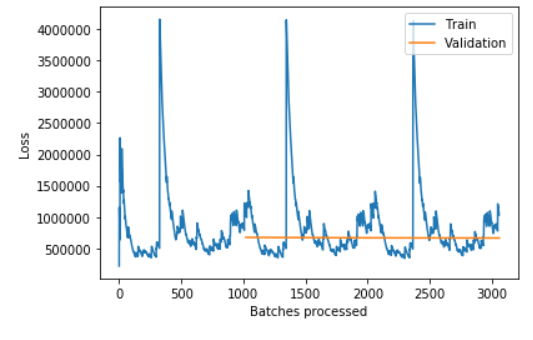# Loss repeats in the same pattern each epoch

I’m trying to train an LSTM on words for regression (to predict numbers). I noticed that the validation loss stays relatively constant, and the training loss spikes up at the beginning of each epoch and goes down to the same level in the same manner each time. Also, when I try to use the learning rate finder and plot the learning rate, it fails and says that it can’t compute the gradient. I’m implementing an LSTM on my own, modelling it after fastai and using the Learner class.

Here is what I’ve done:

``````import torch.nn as nn
class LSTM(nn.Module):

def __init__(self):
super(LSTM, self).__init__()
self.i_h = nn.Embedding(nv,nh)
self.lstm = nn.LSTM(nh, nh, 1)
self.h_o = nn.Linear(nh, 1)
self.bn = BatchNorm1dFlat(nh)
self.dropout = nn.Dropout(0.3)
self.h = torch.zeros(1, bptt, nh).cuda()
self.c = torch.zeros(1, bptt, nh).cuda()

def forward(self, x):
embedded = self.i_h(x)
res, (hn, cn) = self.lstm(embedded, (self.h, self.c))
self.h = hn.detach()
self.c = cn.detach()
out = self.h_o(self.bn(res.view(-1, nh)))
out = self.dropout(out)
out = out.squeeze(1)
out = out.view(bs, bptt)
return out

learn = Learner(data, LSTM())
learn.loss_func=MSELossFlat()
``````

And here is a visualization of three cycles of training: